#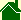The Big Bang (According to the Ball-of-Light Particle Model)

What follows is a new, unique version of the Big Bang. (The Big Bang theory is actually not one theory. There are many variations of the Big Bang.)

If scientists agree that the observational evidence does support the Ball-of-Light Particle Model version of the Big Bang -- the version of the Big Bang described at this website -- then this variation of the Big Bang will usher in a dramatic new Cosmology along with many new philosophical and religious implications.

### The First Ball-of-Light

There is much evidence that the universe is undergoing a massive explosion. Whether this explosion started at a "singularity" or, expanded from a "sphere" of definite size is open to debate. (See also, Inflationary Theory.)

The Ball-of-Light Particle Model predicts the Big Bang explosion started expanding from a preexisting sphere of light of definite size.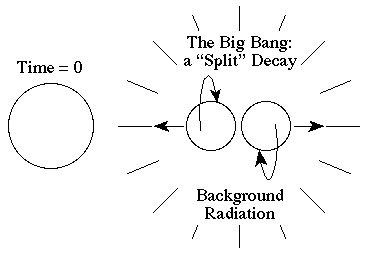At time = 0, this original ball-of-light split into essentially two halves. This original decay certainly released a massive sphere of light as well, and possibly many other smaller balls-of-light, but it essentially left two massive spheres of light.

## Handedness

The energy in the two remaining spheres had angular momentum that added up to zero. Each of the two spheres had opposite angular momentum. Thus, one half of the universe would have motion that, in general, would follow the "right hand rule" and the other half would have motion that, in general, would follow the "left hand rule." (For a visible example of a similar "split" explosion on a smaller scale, see also The Antennae Galaxies)

While energy can follow either the right hand or left hand rule, it has been observed that the energy in our region of the universe primarily follows the right hand rule. We live in a "right-handed" region of the universe. The fact that our region of universe is right-handed has perplexed scientists for years but is predicted by the Ball-of-Light Particle Model. Any decaying ball-of-light will have two halves of oppositely directed angular momentum.

## The Right Hand Rule and the Left Hand Rule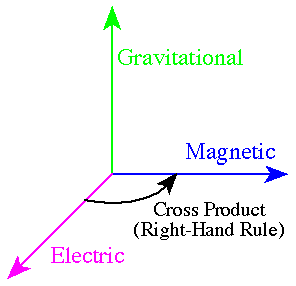The "right hand rule" and the "left hand rule" are simply mathematical conventions of how to multiply two forces. When multiplying two forces a magnitude is obtained but a direction is also needed. The simplest possible mathematical convention describes two oppositely directed vectors. One is called "right-handed," the other is called "left-handed."

To understand the right hand rule, imagine placing your right hand in the image above. Your right arm would be parallel to the electric field. Your fingers would curl towards the magnetic field. Your thumb would be pointing up.

The left hand rule would point your thumb down in this image. It would point in the opposite direction as the right hand rule. Your left arm would be parallel to the electric field. Your fingers would curl towards the magnetic field. Your thumb would be pointing down.

At first, when you do this, you find yourself doing these ridiculous contortions. But after you get used to it, you simply do it in your head.

The multitude of possible variations -- the beautiful symmetries -- of combining the electric, magnetic, and gravitational fields using the Grand Unification Theory called the Ball-of-Light Particle Model is truly amazing. To see all of these possible variations, see also Table A, and Table B.

## How did the original decay start?

The Ball-of-Light Particle Model does not explicitly address the two questions: "How did such a massive sphere of light get there in the first place?"; and, "Why did it explode?" I believe these are religious questions. There is a point where science and logic will always fail and faith is required. The beginning of the universe will always be one such point. The end of the universe will always be another.

(Personally, I view the initial sphere as "God".)

### The Big Crunch

The Ball-of-Light Particle Model predicts that the universe will contract after it finishes expanding. In essence, the electric and magnetic energy of the universe is being converted to gravitational potential energy. (See also, Cepheid Variables) Furthermore, this theory predicts that the universe will eventually collapse and when the universe collapses, it would then explode again, and that it is possible this Big Bang is simply one in a series of Big Bangs and Big Crunches.

### The next two Balls-of-Light

The Ball-of-Light Particle Model predicts that the initial elementary particle -- the Ball-of-Light that contained all of the energy of the universe -- began its explosion by splitting in two.

This "splitting in two" is important. It explains: why our portion of the universe is primarily made of matter, not antimatter (See also, matter versus antimatter); and why our portion of the universe is primarily "right handed" instead of "left handed" (See also, Handedness). (I have already had numerous people point out the interesting religious implications of this splitting in two.)

### The first energy level

This initial explosion that started the Big Bang would be the "first energy level" in a series of energy levels that have a harmonic pattern.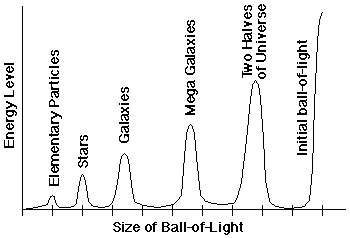It has been found that nature has many natural harmonic patterns at different, widely spaced, energy levels. The graph above highlights the most significant energy levels: the initial ball-of-light, the two halves of the universe, Mega-Galaxies, galaxies, stars, and "normal" elementary particles.

Each energy level, except the first two, appears to have sublevels. For example, one energy level -- that of normal elementary particles -- has many known sublevels. When analyzing the energy released from collisions in particle accelerators, scientists have found that the output energy does not increase smoothly as the input energy is increased, but rather the output energy follows harmonic patterns.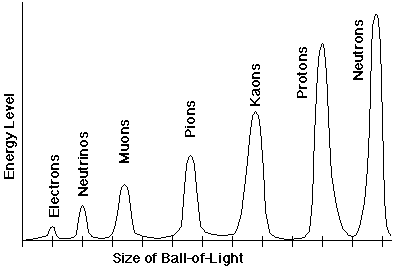Where humps are observed in the curve, scientists attribute the humps to the existence of particles. In many cases, when analyzing the harmonics, scientists have found that the lifetimes of the particles are so fleeting the particles can not actually be obs
erved, only inferred.

In other words, the photons of light combine into an extremely nonharmonic spherical pattern -- the particle's harmonic period is so brief that it decays almost as soon as it is made.

Scientists have also found that if a normally nonharmonic particle is accelerated to high speeds its stability will increase. Traditionally, scientists have explained this with Relativity and "time dilation." The Ball-of-Light Particle Model predicts that high-speed particles will electromagnetically induce stronger center-pointing gravitational fields that will make the particle more stable. This stability is lost however as the particle slows. (See also, Zones of Instability, The Decay of Muons, and Cassiopeia A for examples.)

### The Second Energy Level

The Ball-of-Light Particle Model predicts that when the initial ball-of-light -- that contained all of the energy of the universe -- exploded in the Big Bang, that it split into two balls-of-light. These two balls-of-light then exploded into smaller balls-of-light.

The later explosion of these two halves would be the "second energy level" in a series of energy levels that have a harmonic pattern.